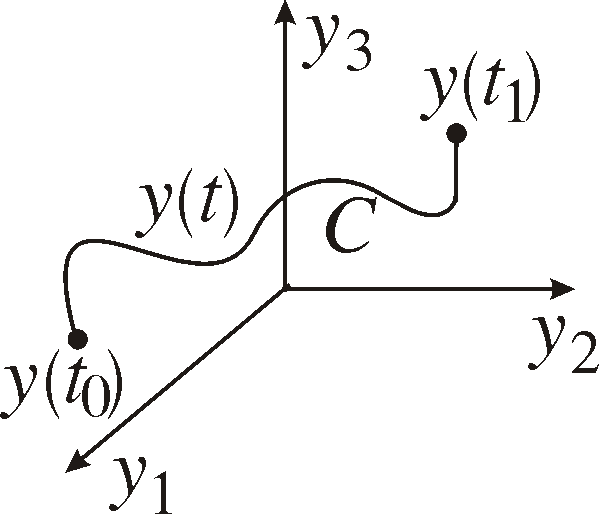1. The Lagrangian

We will in this chapter use calculus of variations to get the equations of motion for a mechanical system. I next part we will see that the motion of the system is such that a specific integral is minimized. Consider a mechanical systemthat is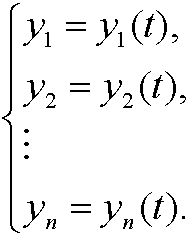Here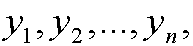are the so called generalized coordinates andare called generalized velocities. If aij are known functions of the coordinates y1,y2,…,yn, we denote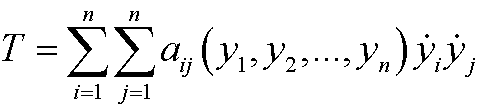the generalized kinetic energy and the generalized potential energy as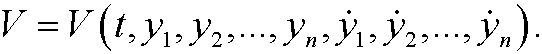We can now define the Lagrangian asIf we consider the general coordinates y1,y2,…,yn, as coordinates in Rn the equations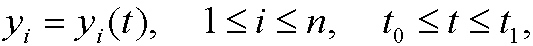can be considered parametric equationes for a curve C connecting two states S0 and S1 in space.